Question

Dynamics

Q3. The beam AB, shown in Figure 3a), has the cross-sectional area as shown in Figure 3b).The loaded beam is a ""-shape steel beam reinforced with a horizontal rectangular-shape wooden beam throughout its length AB (shown in black in Figure 3b). Do the following:

(a) Derive the centroid position from the top of the cross-section, y

(b) Calculate the second moment of area about axis zz for the overall (steel and wooden component) beam cross-section. The second moment of area of the steel section of the beam about axis zz is Isteel=4.24 x 10-5 (m4)[5 marks]

(c) Calculate maximum tensile and compressive bending stresses in the beam at a position8 m away from the support A.[8 marks)

(d) Determine the bending stress at the point located 62 mm above the bottom edge of the cross-section (point C), at a distance of 8 m away from the support A. Also calculate the percentage increase of bending stress at this point if the steel beam was not reinforced with the wooden beam as shown in Figure 3b.[5 marks]Verified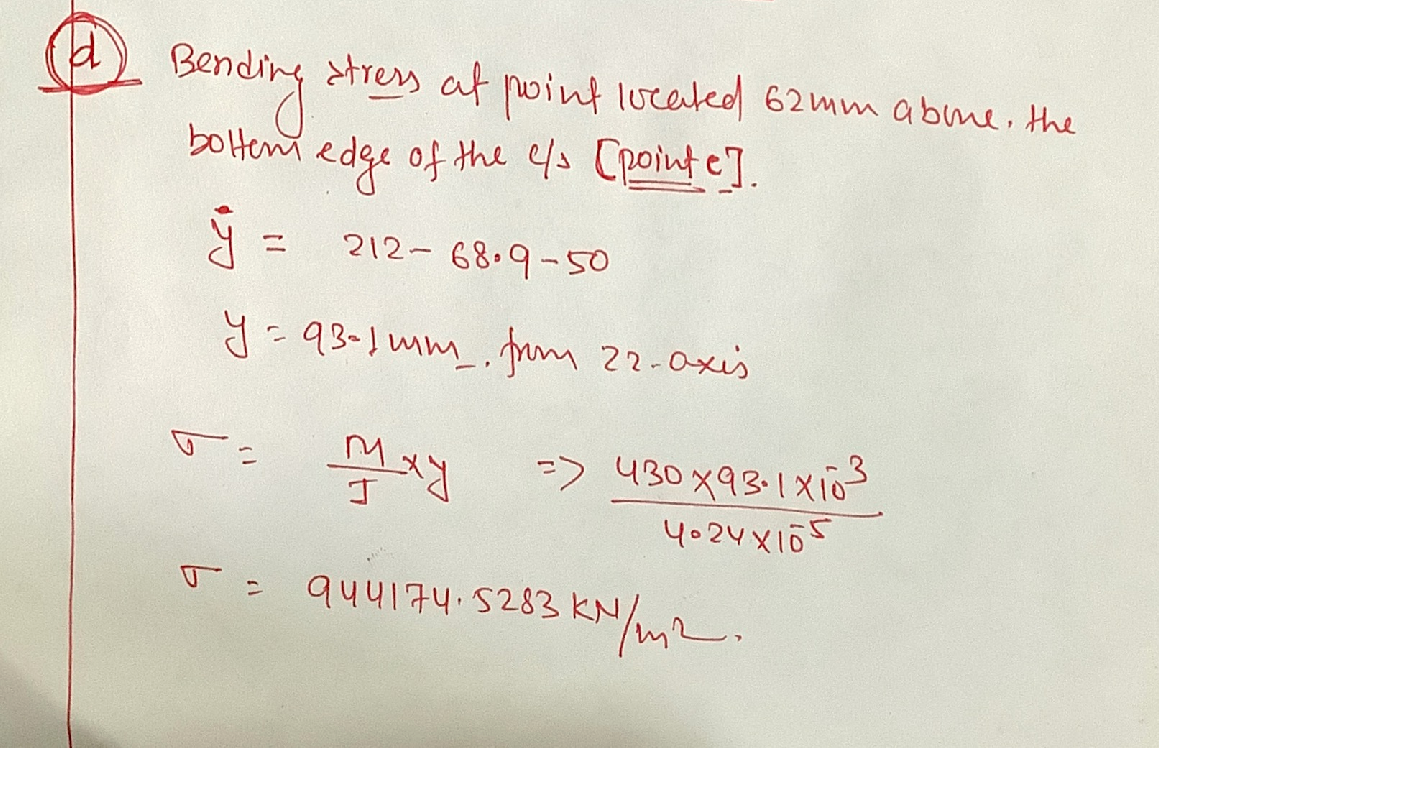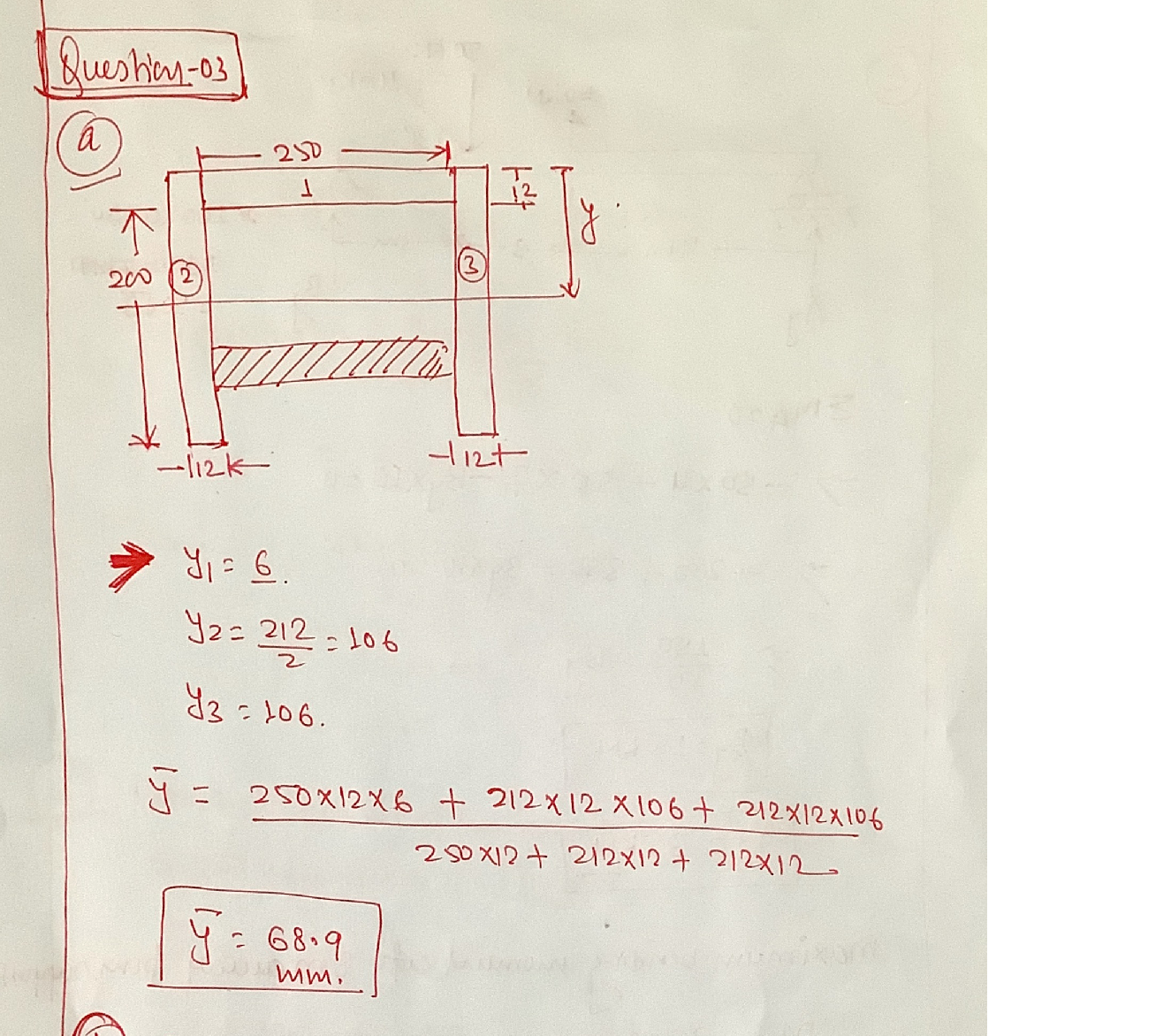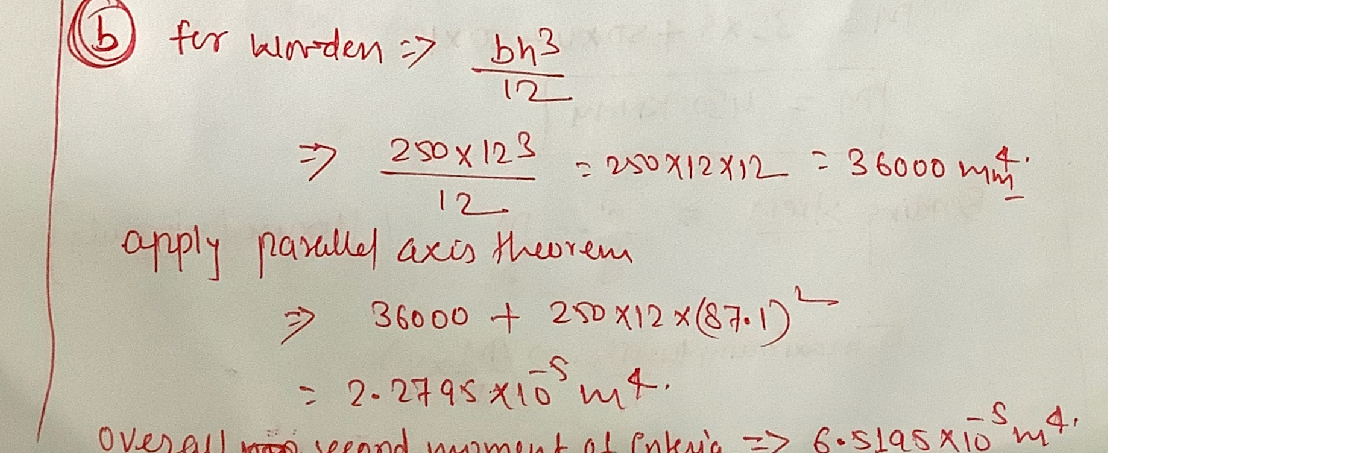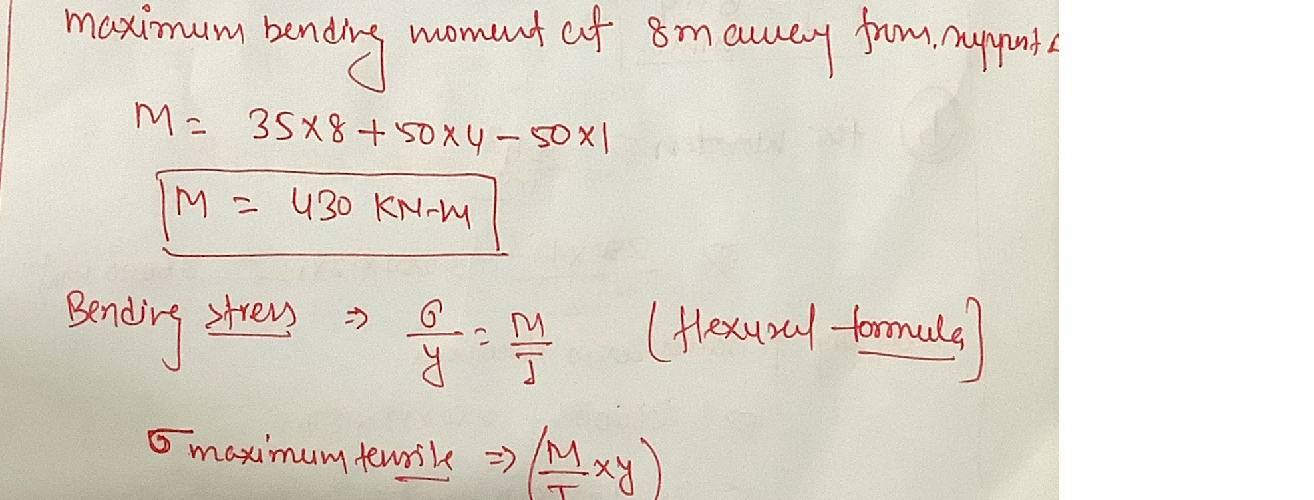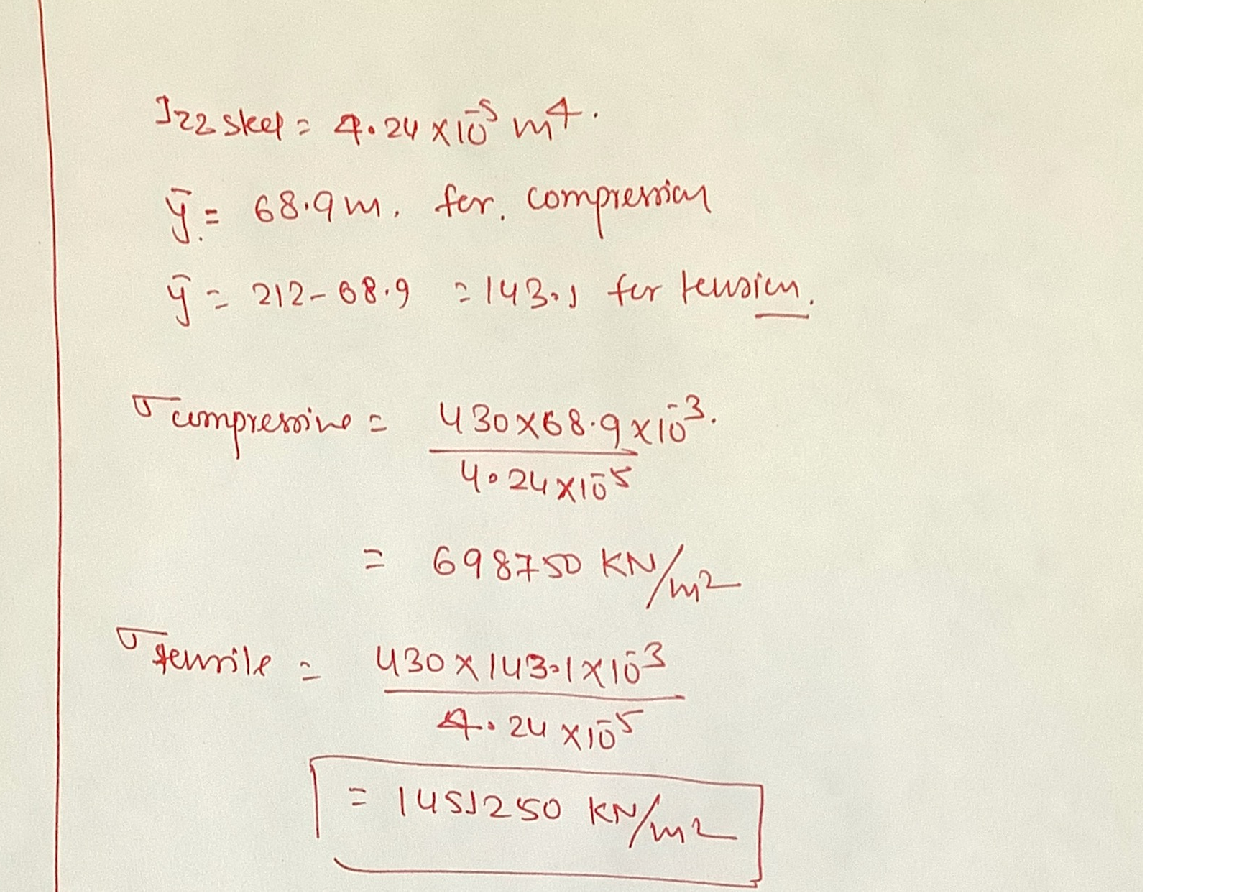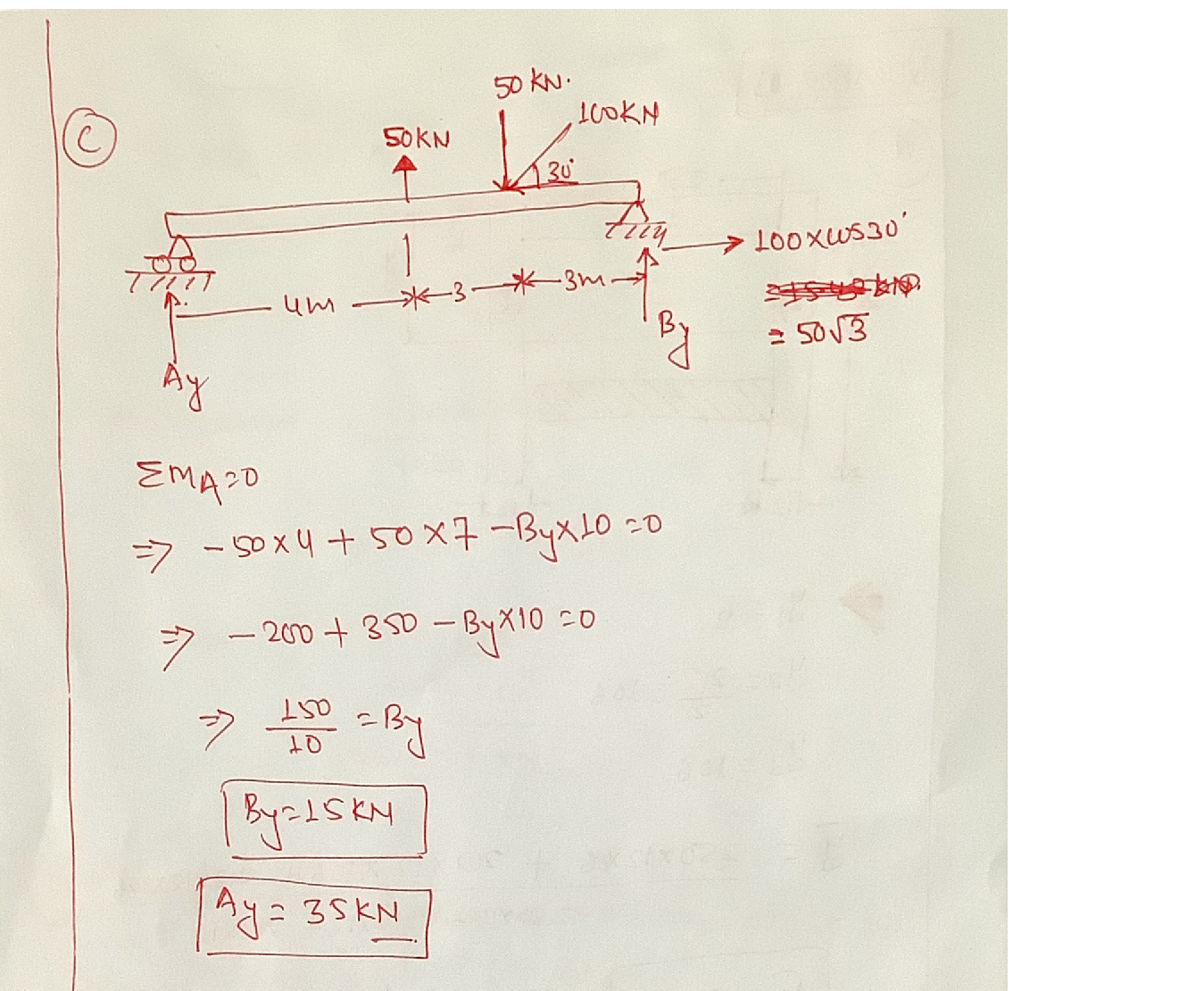### Question 45555Dynamics

*15-40. The boy jumps off the flat car at A with a velocity of v = 4 ft/s relative to the car as shown. If he lands on the second flat car B, determine the final speed of both cars after the motion. Each car has a weight of 80 lb. The boy's weight is 60 lb. Both cars are originally at rest. Neglect the mass of the car's wheels

### Question 45554Dynamics

15-57. The 10-kg block is held at rest on the smooth inclined plane by the stop block at A. If the 10-g bullet is traveling at 300 m/s when it becomes embedded in the10-kg block, determine the distance the block will slide up along the plane before momentarily stopping.

### Question 45553Dynamics

15-54. Solve Prob. 15-53 if the coefficient of kinetic friction between A and B is H = 0.3. Neglect friction between block B and the horizontal plane

### Question 45552Dynamics

15-15. The motor, M, pulls on the cable with a force F=(10f + 300) N, where r is in seconds. If the 100 kg crate's originally at rest at t=0, determine its speed when t=4 s Neglect the mass of the cable and pulleys. Hint: First find the time needed to begin lifting the crate.

### Question 45551Dynamics

15-22. The thrust on the 4-Mg rocket sled is shown in the graph. Determine the sleds maximum velocity and the distance the sled travels when t 35 s. Neglect friction.

### Question 45550Dynamics

15-10. The 50-kg crate is pulled by the constant force P. Ifthe crate starts from rest and achieves a speed of 10 m/s in5 s, determine the magnitude of P. The coefficient of kinetic friction between the crate and the ground is Hi =0.2.

### Question 45549Dynamics

*15-8. The automobile has a weight of 2700 lb and is-traveling forward at 4 ft/s when it crashes into the wall. Ifthe impact occurs in 0.06 s, determine the average impulsive force acting on the car. Assume the brakes are not applied. If the coefficient of kinetic friction between the wheels and the pavement is H = 0.3, calculate the impulsive force on the wall if the brakes were applied during the crash.The brakes are applied to all four wheels so that all the wheels slip.

### Question 45467Dynamics

As a part of a robotics competition, a robotic arm with a rigid open hand at C is to be designed so that the hand catches an egg without breaking it. The egg is released from rest at t = 0 from point A. The arm, initially at rest in the position shown, starts moving when the egg is released. The hand must catch the egg without any impact with the egg. This can be done by specifying that the hand and the egg must be at the same position at the same time with identical velocities. A student proposes to do this using a constant value of 0 for which (after a fair bit of work) it is found that the arm catches the egg at /= 0.4391 s forÖ = -13.27 rad/s2. Using these values of t and Ö, determine the acceleration of both the hand and the egg at the time of catch.Then, explain whether or not using a constant value of 0, as has been proposed, is an acceptable strategy.

### Question 45466Dynamics

A crate A is being pulled up an inclined ramp by a winch retracting the cord at a constant rate vo = 2 ft/s. Letting h =1.5 ft,determine the speed of the crate when d = 4 ft.

### Question 45463Dynamics

At the instant shown, VB = 5 î m/s. If theta = 25°, determine the speed of A relative to B in order for A to travel only in the vertical direction while sliding over B.

### Submit query

Getting answers to your urgent problems is simple. Submit your query in the given box and get answers Instantly.

### Submit a new QuerySuccess

Assignment is successfully created